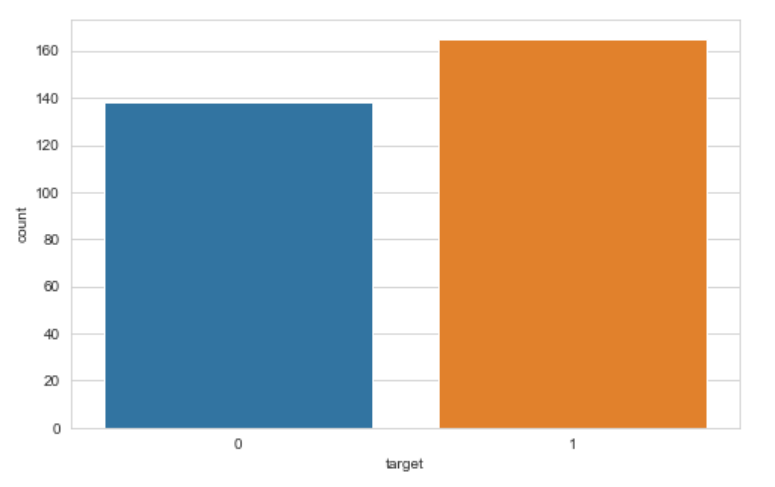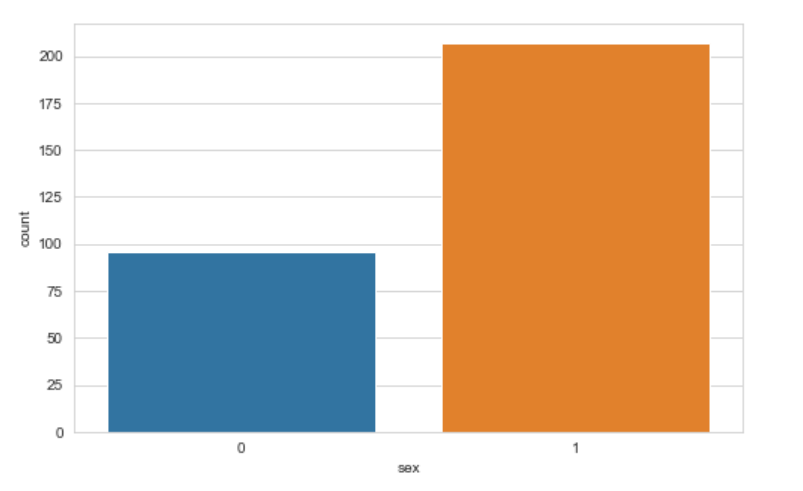top of page
Search

# Heart Disease data set - classification

Updated: Nov 3, 2021## Description :

The heart disease dataset is available on kaggle and UCI Machine learning Repository. According to UCI, "This dataset contains 76 attributes, but all published experiments refer to using a subset of 14 of them. In particular, the Cleveland database is the only one that has been used by ML researchers to this date." We can use this dataset for classification, to predict whether patients have heart disease by giving some features of users.

## Recommended Model :

Algorithms to be used, Logistic Regression, SVM, Naive Bayes, Random Forest, Neural network etc.

## Recommended Projects :

To predict whether patients have heart disease by giving some features of users.

Data set Link : Kaggle : - https://www.kaggle.com/ronitf/heart-disease-uci

## Overview of data

Detailed overview of dataset

• Records in the dataset = 303ROWS

• Columns in the dataset = 14 COLUMNS

1. Age : -Patient’s age in year (continuous value)

2. Sex : - Gender of Patient (1- male , 0- femae )

3. CP : Chest Pain (1- typical angina, 2- atypical angina, 3 - non- angina pain, 4- asymptomatic)

4. Trestbps : - Resting Blood Pressure (continuous value in mm/hg)

5. Chol : - serum cholesterol in mg/dl (continuous value mg/dl)

6. FBS :- Fasting Blood sugar

7. Restege : Resting Electrographic result

8. Thalach : -Maximum heart rate achieved (continuous value)

9. Exang : Exercise induced angina

10. Oldpeak : ST depression induced by exercise relative to rest

11. Slope : the slope of the peak exercise ST segment

12. Ca : number of major vessels coloured by fluoroscopy

13. Thal : defect type

14. Num : diagnosis of heart disease

## EDA[Code]

Dataset

```import pandas as pd
file_loc = "data\\heart.csv"Total number of rows and columns

```# Number of Rows and columns
rows_col = heart_data.shape
print("Total number of Rows in the dataset : {}".format(rows_col))
print("Total number of columns in the dataset : {}".format(rows_col))

```Check Details

```# Data information
heart_data.info()```Check Missing values

```# Missing Values
heart_data.isna().sum()```Statistical information

```# Statistical information
heart_data.describe()```Data Visualization

Correlation

```import seaborn as sns
import matplotlib.pyplot as plt
# correlation
corr = heart_data.corr()correlation

Count the heart patient

```# 0 means no, 1 - yes
sns.set_style("whitegrid")
plt.figure(figsize=(8,5))
sns.countplot(x= "target",data=heart_data)```target

Count the number of male and female patient

```# Gender Male and Female
plt.figure(figsize=(8,5))
sns.countplot(x= "sex",data=heart_data)```Count plot of chest pain

```# Chest pain
plt.figure(figsize=(8,5))
sns.countplot(x= "cp",data=heart_data)```chest pain count

count plot of fast blood pressure

```# fasting blood pressure
plt.figure(figsize=(8,5))
sns.countplot(x= "fbs",data=heart_data)```fast blood pressure count

Count plot of Resting Electorgraphic result

```# resting electrocardiographic results
plt.figure(figsize=(8,5))
sns.countplot(x= "restecg",data=heart_data)```Resting electrographic result count

Exercise induced angina count

```# exercise induced angina 0 - no  1 - yes
plt.figure(figsize=(8,5))
sns.countplot(x= "exang",data=heart_data)```Exercise induced angina count

Count plot of thal

```plt.figure(figsize=(8,5))
sns.countplot(x= "thal",data=heart_data)```Thal count

Histogram plot of age

```# Histogram
plt.figure(figsize=(8,5))
sns.histplot(x="age",data=heart_data)```Age histogram

## Other related data

Occupancy Detection Data Set - Classification

Census income Data Set - Classification

Wholesale customer - Classification and Clustering

Online retail dataset - classification, clustering and regression

Cervical Cancer Risk Factor Dataset - classification and clustering

Blood Transfusion service center dataset - Classification

Divorce Predictor Dataset -classification

Fire Forest Dataset - Regression

Student performance dataset - Classification and Regression

If you need implementation for any of the topics mentioned above or assignment help on any of its variants, feel free to contact us.bottom of page